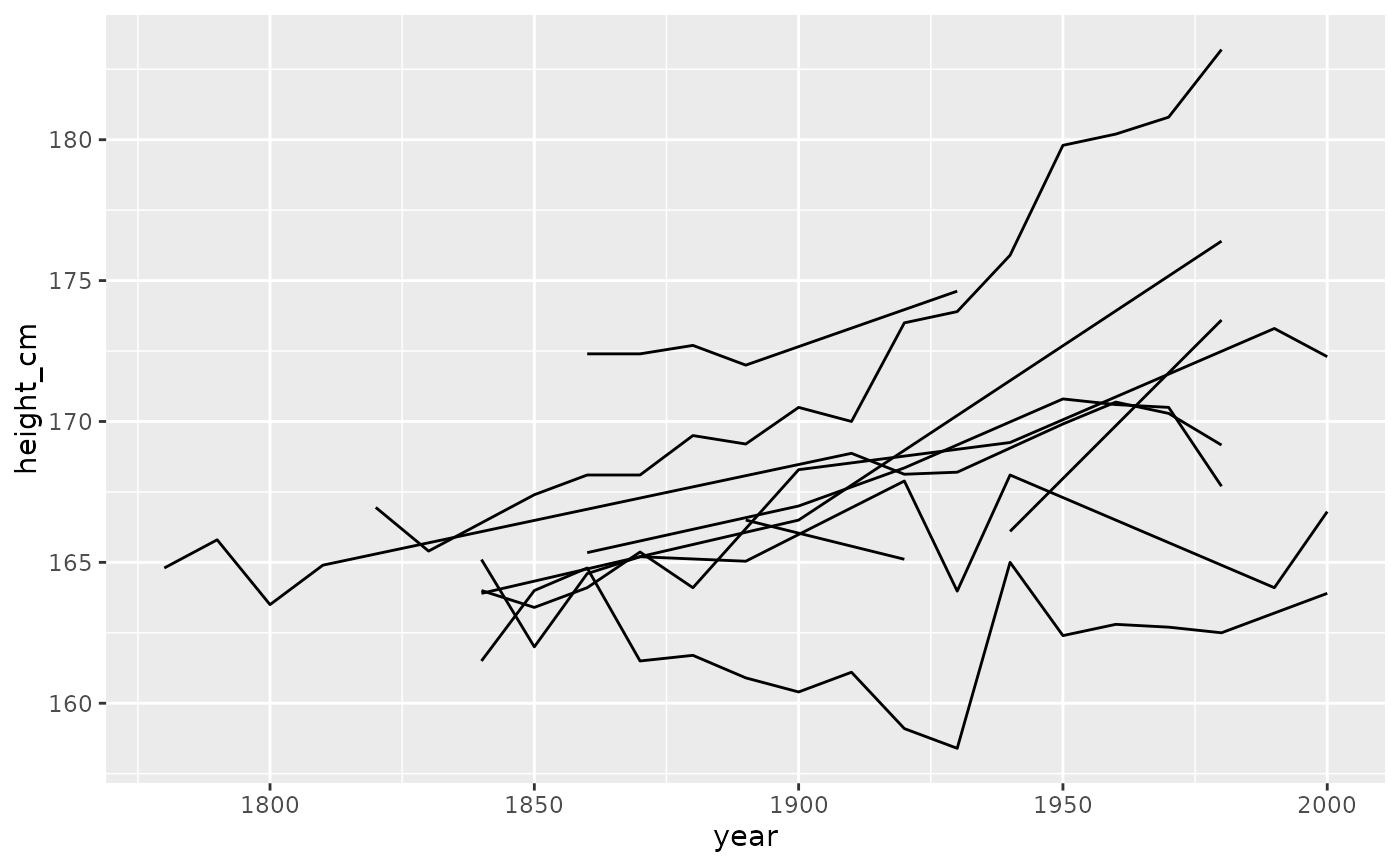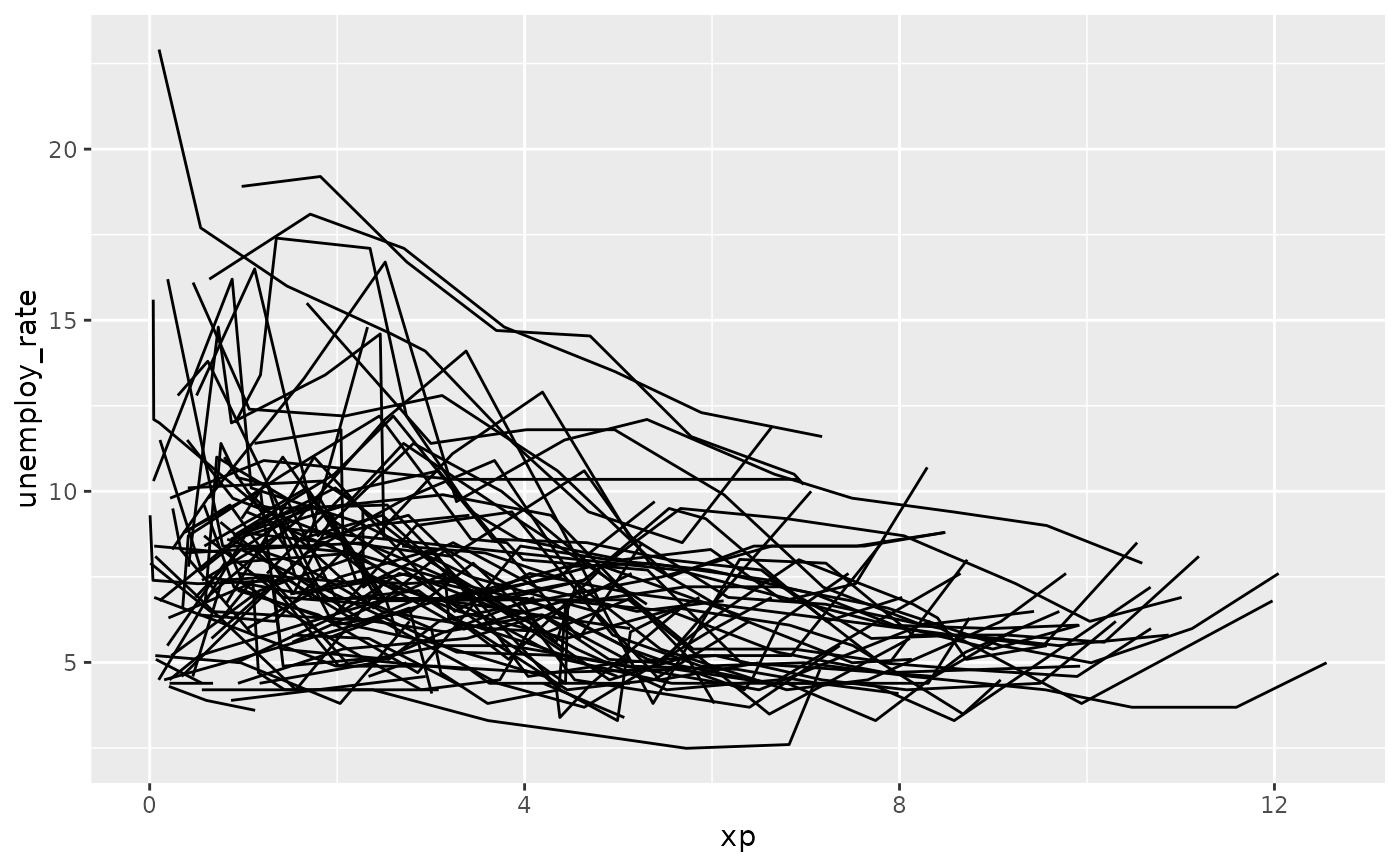Sample a number or fraction of keys to explore

sample_n_keys(.data, size)

sample_frac_keys(.data, size)

## Arguments

.data tsibble object The number or fraction of observations, depending on the function used. In sample_n_keys, it is a number > 0, and in sample_frac_keys it is a fraction, between 0 and 1.

## Value

tsibble with fewer observations of key

## Examples

library(ggplot2)
sample_n_keys(heights,
size = 10) %>%
ggplot(aes(x = year,
y = height_cm,
group = country)) +
geom_line()library(ggplot2)
sample_frac_keys(wages,
0.1) %>%
ggplot(aes(x = xp,
y = unemploy_rate,
group = id)) +
geom_line()